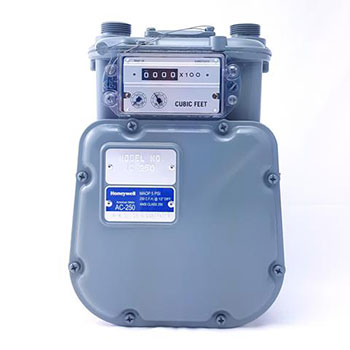#### Reading Your Gas MeterGas meters measure the amount of gas used by counting the filling and emptying of compartments inside the meter. To find out how much gas was used over a certain period of time - such as one day or a billing period - take a "reading" at the beginning of the period and again at the end. The difference between the two readings is the amount of gas used.

Residential gas meters have one or two dials marked 10 feet or less. These should not be read. They are for testing only.

The dials that record the amount of gas used are the three, four, or sometimes five - grouped together - that are marked "1 thousand" and over. (Notice that each dial is numbered in the opposite direction from the dial next to it.)

Although your meter dials may not look quite like the ones here, these instructions can be used to read most residential meters. Each dial represents a single number in the reading.Read the meter dials right to left, writing down the numbers in the same order - right to left. If the hand points between two numbers, always use the lower number. (When a hand points between nine and zero, it will always be read as a nine.)

When the hand seems to be directly on a number, look at the dial to the right. If the hand on that dial is on or just past zero, write down the number the hand is pointing toward on the dial you are reading.

If the hand on the dial to the right hasn't reached zero, write down the smaller number on the dial you are reading. When reading the far right-hand dial, write down the number the hand appears to be pointing toward, because there is no dial to the right to check.

The correct reading for this meter is 1378. This means 1378 hundred cubic feet (CCF) of gas has passed through the meter since all dials were on zero. Another way to write this amount is 137,800 cubic feet.

To compute your gas bill, the number of CCFs* used during the current billing period is multiplied by the current Btu factor to determine the number of therms used.

(*CCF is a measure of the volume of gas used, while the Btu factor tells how much heat each CCF of gas contains. The Btu factor is a characteristic of the gas and may vary a little from month to month.)